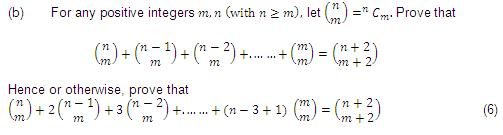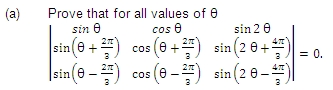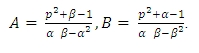×#### Thank you for registering.

One of our academic counsellors will contact you within 1 working day.

Click to Chat

1800-1023-196

+91-120-4616500

CART 0

• 0

MY CART (5)

Use Coupon: CART20 and get 20% off on all online Study Material

ITEM
DETAILS
MRP
DISCOUNT
FINAL PRICE
Total Price: Rs.

There are no items in this cart.
Continue Shopping• Complete JEE Main/Advanced Course and Test Series
• OFFERED PRICE: Rs. 15,900
• View Details

```IIT-JEE-Mathematics-Mains–2000

MAINS

Time : two hours                                                                  Max. Marks : 100
_____________________________________________________________________
General Instructions :
1. There are ten questions in this paper. Attempt all Questions.
2. Answer each question starting on a new page. The corresponding question number must be written in the left margin. Answer all the parts of a question at one place only.
3. Use only Arabic numerals (0, 1, 2 ………….9) in answering the questions irrespective of the language in which your answer.
4. Use of logarithmic tables is not permitted.
5. Use of calculator is not permitted.
_____________________________________________________________________
PART- B

1. (a) The fourth power of the common difference of an arithmetic progression with integer entries is added to the product of any four consecutive terms of it. Prove that resulting sum is the square of an integer.                                                           (4)2. (a) If α, β are the roots of ax2+bx+c=0,(a≠0) and α+d, β+d are the roots of Ax2+Bx+C=0,(A≠0)for some constant d, then prove that
(b2-4ac)/a2 =(B2-4AC)/A2                                                              (4)

(b) For every positive integer, prove that
√(4n+1)  <  √n + √(n+1)  <   √(4n+2)
Hence or otherwise, prove that [√n+√(n+1) = [√(4n+1]), where [x] denotes the greatest integer not exceeding x.                                                    (6)

3. (a) In any triangle ABC, prove that
Cot A/b + cot B/2 + cot C/2 = A/2 cot B/2 cot C/2                             (3)

(b) Let ABC be a triangle with incentre I and inradius r. Let D, E, F be the feet of the perpendiculars from I to the sides BC, CA and AB respectively. If r1, r2 and r3 are the radii of circles inscribed in the quadrilaterals AFIE, BDIF and CEID respectively, prove that
r1/ (r - r1 ) + r2 / (r - r2) + r3  / (r - r3) = (r1 r2 r3 ) / (r - r1) (r-r2) (r-r3)

4. For points P = (x1, y1) and Q = (x2, y2) of the coordinate plane, a new distance d(P, Q) is defined by d (P, Q) = ¦x1–x2¦+¦y1–y2¦. Let O = (0, 0) and A=(3, 2). Prove that the set of points in the first quadrant which are equidistant (with respect to the new distance) from O and A consists of the union of line segment of finite length and an infinite ray. Sketch this set in a labelled diagram.

5.(b) Let ABC be an equilateral triangle inscribed in the circle x2 + y2 = a2. Suppose perpendiculars from A, B, C to the major axis of the ellipse x2/a2 +y2/b2 =1, (a>b) meets the ellipse respectively at P, Q, R so that P, Q, R lie on the same side of the major axis as A, B, C respectively. Prove that the normals to the ellipse drawn at the points P, Q and R are concurrent.

6. Let C1 and C2 be, respectively, the parabolas x2 = y – 1 and y2 =  a2. Let P be any point on C1 and Q be any point on C2. Let P1 and Q1 be the reflections of P and Q, respectively, with respect to the line y = x. Prove that P1 lies on C2, Q1 lies on C1and PQ ≥ min {PP1, QQ1}. Hence or otherwise, determine points P0 and Q0 on the parabolas C1 and C2 respectively such that P0 Q0 ≤ P0 for all pairs of points (P, Q) with P on C1 and Q on C2.

7.8. A country has food deficit of 10%. Its population grows continuously at a rate of 3% per year. Its annual food production every year is 4% more than that of the last year. Assuming that the average food requirement per person remains constant, prove that the country will become self-sufficient in food after n years, where n is the smallest integer bigger than
or equal to    (In 10 - In 9) / (In (1.04)- 0.03).

9. (a) A coin has probability p of showing head when tossed. It is tossed n times. Let pn, denote the probability that no two (or more) consecutive heads occur. Prove that p1 = 1, p2 = 1 – p2 and    pn = (1 – p). pn–1 + p (1 – p) pn – 2 for all n ≥ 3.

(b) In (a), prove by induction on n, that pn = A αn + Bβn for all n ≥ 1, where α and β are the roots of the quadratic x2 – (1 – p) x – p (1 – p) = 0 and10. Let ABC and PQR be any two triangles in the same plane. Assume that the perpendiculars from the points A, B, C to the sides QR, RP, PQ respectively are concurrent. Using vector methods or otherwise, prove that the perpendiculars from P, Q, R to BC, CA, AB respectively are also concurrent.
```### Course Features

• 731 Video Lectures
• Revision Notes
• Previous Year Papers
• Mind Map
• Study Planner
• NCERT Solutions
• Discussion Forum
• Test paper with Video Solution